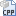# Find the HCF and LCM by using the C++

### Find the HCF and LCM by using the C++

#include using namespace std;
main(){
int a,b,c;
cout"Enter Two Numbers...\n\n";
cout"Enter Number 1 :";
cin>>a;
cout"Enter Number 2 :";
cin>>b;
c=a*b;
while(a!=b){
if(a>b){
a=a-b;
}
else{
b=b-a;
}
}
cout"HCF :"a>"lcm="" :="" "c="" aendl;="" couten...

if the above code does not work then

Attachments:

## C++ Tutotial #2

### WRITE A C++ PROGRAM TO FIND LCM OF TWO NUMBERS

```# include <iostream>
# include <string >
using namespace std;
int main()
{
int a,b,c;
cout<< "Enter two nos :"<<endl;
cout<<endl;
cout<< "Enter first no. : ";
cin>>a;
cout<< "Enter sec. no. : ";
cin>>b;
c=a*b;
while(a!=b)
{
if(a>b)
a=a-b;
else
b=b-a;
}
cout<< "HCF = " << a<<endl;
cout<< "LCM = " << c/a<<endl;
return 0;
}```